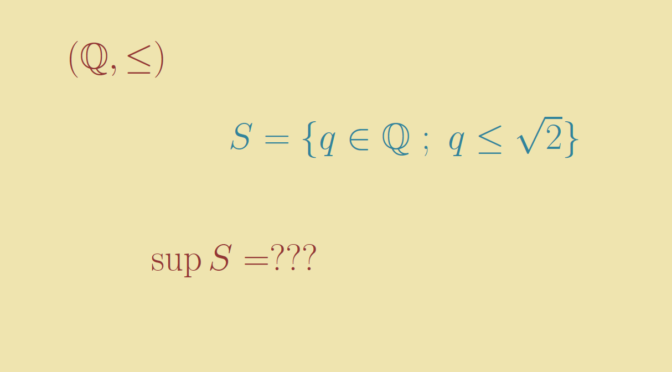Around bounded sets, greatest element and supremum

Consider a linearly ordered set $$(X, \le)$$ and a subset $$S \subseteq X$$. Let’s recall some definitions:

• $$S$$ is bounded above if there exists an element $$k \in X$$ such that $$k \ge s$$ for all $$s \in S$$.
• $$g$$ is a greatest element of $$S$$ is $$g \in S$$ and $$g \ge s$$ for all $$s \in S$$. $$l$$ is a lowest element of $$S$$ is $$l \in S$$ and $$l \le s$$ for all $$s \in S$$.
• $$a$$ is an supremum of $$S$$ if it is the least element in $$X$$ that is greater than or equal to all elements of $$S$$.

Subsets with a supremum but no greatest element

Let’s give examples of subsets having a supremum but no greatest element. First consider the ordered set $$(\mathbb R, \le)$$ and the subset $$S=\{ q \in \mathbb Q \ ; \ q \le \sqrt{2}\}$$. $$S$$ is bounded above by $$2$$. $$\sqrt{2}$$ is a supremum of $$S$$ as we have $$q \le \sqrt{2}$$ for all $$q \in S$$ and as for $$b < \sqrt{2}$$, it exists $$q \in \mathbb Q$$ such that $$b < q < \sqrt{2}$$ because $$\mathbb Q$$ is dense in $$\mathbb R$$. However $$S$$ doesn't have a greatest element because $$\sqrt{2}$$ is an irrational number.

For our second example we take $$X = \mathbb N \times \mathbb N$$ ordered lexicographically by $$\preceq$$. The subset $$S=\{(0,n) \ ; \ n \in \mathbb N\}$$ is bounded above by $$(2,0)$$. Moreover $$(1,0)$$ is a supremum. But $$S$$ doesn’t have a greatest element as for $$(0,n) \in S$$ we have $$(0,n) \prec (0,n+1)$$.

Bounded above subsets with no supremum

Leveraging the examples above, we take $$(X, \le) = (\mathbb Q, \le)$$ and $$S=\{ q \in \mathbb Q \ ; \ q \le \sqrt{2}\}$$. $$S$$ is bounded above, by $$2$$ for example. However $$S$$ doesn’t have a supremum because $$\sqrt{2} \notin \mathbb Q$$.

Another example is the set $$X = \mathbb N \times \mathbb Z$$ ordered lexicographically by $$\preceq$$. The subset $$S=\{(0,n) \ ; \ n \in \mathbb N\}$$ is bounded above by $$(2,0)$$ but has no supremum. Indeed, the elements greater or equal to all the elements of $$S$$ are the elements $$(a,b)$$ with $$a \ge 1$$. However $$(a,b)$$ with $$a \ge 1$$ cannot be a supremum of $$S$$, as $$(a,b-1) \prec (a,b)$$ and $$(a,b-1)$$ is greater than all the elements of $$S$$.